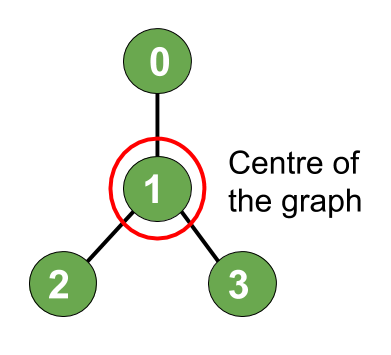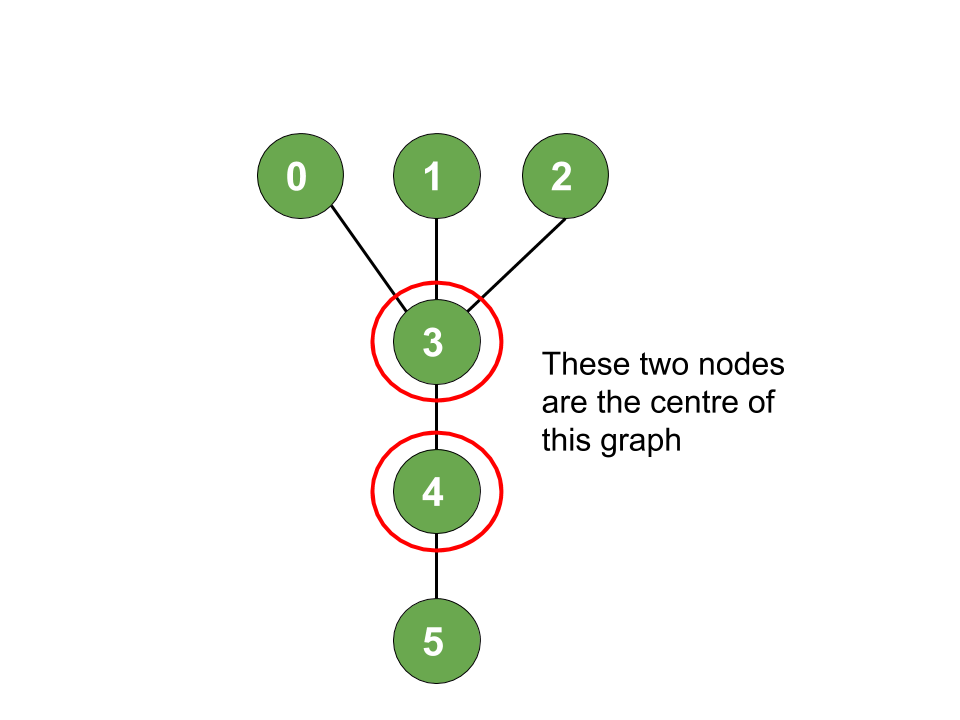# Find the node at the centre of an N-ary tree

Prerequisites:

Given a N-ary tree with N nodes numbered from 0 to N-1 and a list of undirected edges, the task is to find the node(s) at the centre of the given tree.

Eccentricity: The eccentricity of any vertex V in a given tree is the maximum distance between the given vertex V and any other vertex of the tree.

Centre: The centre of a tree is the vertex having the minimum eccentricity. Hence, it means that in order to find the centre we have to minimise this eccentricity.

Examples:

Input: N = 4, Edges[] = { (1, 0), (1, 2), (1, 3)}
Output: 1
Explanation:Input: N = 6, Edges[] = { (0, 3), (1, 3), (2, 3), (4, 3), (5, 4)}
Output: 3, 4
Explanation:## Recommended: Please try your approach on {IDE} first, before moving on to the solution.

Approach: It can be observed that the path of maximum eccentricity is the diameter of the tree. Hence, the centre of the tree diameter will be the centre of the tree as well.

Proof:

• For example, Let’s consider a case where the longest path consists of odd number of vertices. Let the longest path be X —— O ——– Y where X and Y are the two endpoints of the path and O is the middle vertex.
• For a contradiction, if the centre of the tree is not O but some other vertex O’, then at least one of the following two statements must be true.

1. Path XO’ is strictly longer than path XO
2. Path YO’ is strictly longer than path YO
• This means O’ will not satisfy the condition of minimum eccentricity. Hence by contradiction, we have proved that the centre of the tree is actually the centre of the diameter path.

• Now if the diameter consists odd number of nodes, then there exists only 1 centre (also known as Central Tree).
• If diameter consists of even number of nodes, then there are 2 centre nodes(also known as Bi-central Tree).

Below is the implementation of the above approach:

## C++

 `// C++ implementation of ` `// the above approach ` ` `  `#include ` `using` `namespace` `std; ` ` `  `// To create tree ` `map<``int``, vector<``int``> > tree; ` ` `  `// Function to store the path ` `// from given vertex to the target ` `// vertex in a vector path ` `bool` `getDiameterPath(``int` `vertex, ` `                     ``int` `targetVertex, ` `                     ``int` `parent, ` `                     ``vector<``int``>& path) ` `{ ` ` `  `    ``// If the target node is found, ` `    ``// push it into path vector ` `    ``if` `(vertex == targetVertex) { ` ` `  `        ``path.push_back(vertex); ` `        ``return` `true``; ` `    ``} ` ` `  `    ``for` `(``auto` `i : tree[vertex]) { ` ` `  `        ``// To prevent visiting a ` `        ``// node already visited ` `        ``if` `(i == parent) ` `            ``continue``; ` ` `  `        ``// Recursive call to the neighbours ` `        ``// of current node inorder ` `        ``// to get the path ` `        ``if` `(getDiameterPath(i, targetVertex, ` `                            ``vertex, path)) { ` `            ``path.push_back(vertex); ` `            ``return` `true``; ` `        ``} ` `    ``} ` ` `  `    ``return` `false``; ` `} ` ` `  `// Function to obtain and return the ` `// farthest node from a given vertex ` `void` `farthestNode(``int` `vertex, ``int` `parent, ` `                  ``int` `height, ``int``& maxHeight, ` `                  ``int``& maxHeightNode) ` `{ ` ` `  `    ``// If the current height is maximum ` `    ``// so far, then save the current node ` `    ``if` `(height > maxHeight) { ` `        ``maxHeight = height; ` `        ``maxHeightNode = vertex; ` `    ``} ` ` `  `    ``// Iterate over all the neighbours ` `    ``// of current node ` `    ``for` `(``auto` `i : tree[vertex]) { ` `        ``// This is to prevent visiting ` `        ``// a already visited node ` `        ``if` `(i == parent) ` `            ``continue``; ` ` `  `        ``// Next call will be at 1 height ` `        ``// higher than our current height ` `        ``farthestNode(i, vertex, ` `                     ``height + 1, ` `                     ``maxHeight, ` `                     ``maxHeightNode); ` `    ``} ` `} ` ` `  `// Function to add edges ` `void` `addedge(``int` `a, ``int` `b) ` `{ ` `    ``tree[a].push_back(b); ` `    ``tree[b].push_back(a); ` `} ` ` `  `void` `FindCentre(``int` `n) ` `{ ` `    ``// Now we will find the 1st farthest ` `    ``// node from 0(any arbitary node) ` ` `  `    ``// Perform DFS from 0 and update ` `    ``// the maxHeightNode to obtain ` `    ``// the farthest node from 0 ` ` `  `    ``// Reset to -1 ` `    ``int` `maxHeight = -1; ` ` `  `    ``// Reset to -1 ` `    ``int` `maxHeightNode = -1; ` ` `  `    ``farthestNode(0, -1, 0, maxHeight, ` `                 ``maxHeightNode); ` ` `  `    ``// Stores one end of the diamter ` `    ``int` `leaf1 = maxHeightNode; ` ` `  `    ``// Similarly the other end of ` `    ``// the diameter ` ` `  `    ``// Reset the maxHeight ` `    ``maxHeight = -1; ` `    ``farthestNode(maxHeightNode, ` `                 ``-1, 0, maxHeight, ` `                 ``maxHeightNode); ` ` `  `    ``// Stores the second end ` `    ``// of the diameter ` `    ``int` `leaf2 = maxHeightNode; ` ` `  `    ``// Store the diameter into ` `    ``// the vector path ` `    ``vector<``int``> path; ` ` `  `    ``// Diamter is equal to the ` `    ``// path between the two farthest ` `    ``// nodes leaf1 and leaf2 ` `    ``getDiameterPath(leaf1, leaf2, ` `                    ``-1, path); ` ` `  `    ``int` `pathSize = path.size(); ` ` `  `    ``if` `(pathSize % 2) { ` `        ``cout << path[pathSize / 2] ` `             ``<< endl; ` `    ``} ` `    ``else` `{ ` `        ``cout << path[pathSize / 2] ` `             ``<< ``", "` `             ``<< path[(pathSize - 1) / 2] ` `             ``<< endl; ` `    ``} ` `} ` ` `  `// Driver Code ` `int` `main() ` `{ ` ` `  `    ``int` `N = 4; ` `    ``addedge(1, 0); ` `    ``addedge(1, 2); ` `    ``addedge(1, 3); ` ` `  `    ``FindCentre(N); ` ` `  `    ``return` `0; ` `} `

Output:

```1
```

Time Complexity: O(N)
Auxiliary Space: O(N)

Attention reader! Don’t stop learning now. Get hold of all the important DSA concepts with the DSA Self Paced Course at a student-friendly price and become industry ready.

My Personal Notes arrow_drop_upCheck out this Author's contributed articles.

If you like GeeksforGeeks and would like to contribute, you can also write an article using contribute.geeksforgeeks.org or mail your article to contribute@geeksforgeeks.org. See your article appearing on the GeeksforGeeks main page and help other Geeks.

Please Improve this article if you find anything incorrect by clicking on the "Improve Article" button below.